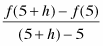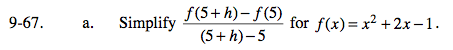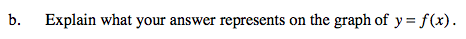### Home > AC > Chapter 16 > Lesson 16.9.2.1 > Problem9-67

9-67.
1. Complete the following. Homework Help ✎

1. Simplifyfor f(x) = x2 + 2x − 1.

2. Explain what your answer represents on the graph of y = f(x).$\frac{(5 + h)^2 + 2(5 + h) - 1 - (5^2 + 2(5) - 1)}{h}$This is a slope expression.